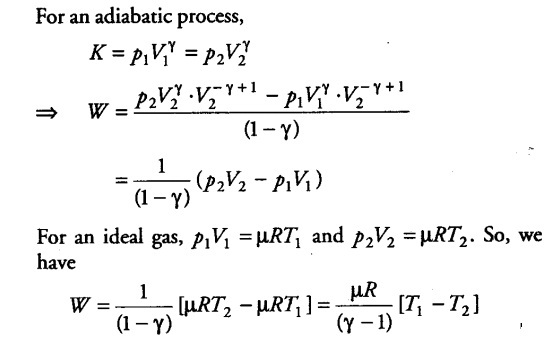# Calculate the work done for adiabatic expansion of a gas

Consider (say (µ mole) an ideal gas, which is undergoing an adiabatic expansion. Let the gas expands by an infinitesimally small volume dV, at pressure p, then the infinitesimally small work done given by
dW = pdV
The net work done from an initial volume { V }_{ 1 } to final volume
{ V }_{ 2 } is given by